[TOC]

# 1. Introduction to substring search

"most ingenious algorithm we've seen so far"
pb. having two strings, `pattern` and `text`, len(pattern)=M << len(text)=N, try to find pattern in text.

ex. `indexOf` method of String in java.

# 2. Brute-Force Substring Search

function signature:
`public static int search(String pat, String txt);`

brute-force algo: look for `pattern` at every position of `text`.

```public static int search(String pat, String txt){
int N=txt.length(), M=pat.length();
for(int i=0; i<=N-M; i++){
int j;
for(j=0; j<M && pat.charAt(j)==txt.charAt(i+j); j++);
if(j==M) return i;
}
return N;// not found
}
```

worst case: when txt/pat are repetitive → MN compares.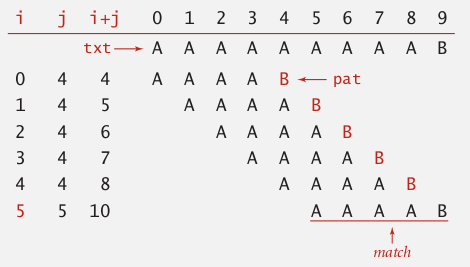problem with brute-force: always backup when mismatch.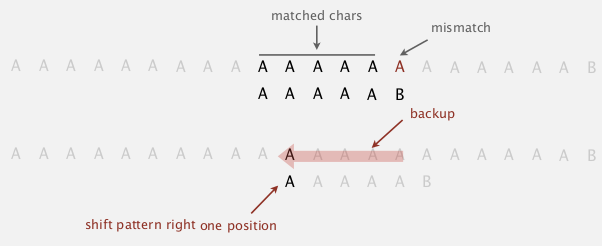brute-force alternative

• j := number of matched chars in pattern
• i := index of the end of matched char in text

⇒ do explicite backup when mismatch by `i -= j`

```public static int search(String pat, String txt){
int N=txt.length(), M=pat.length(), i=0, j=0;
while(i<N && j<M){
if(pat.charAt(j)==txt.charAt(i))
{j=i++; j++;}
else
{i=i-j+1; j=0}// <==backup
}
return j==M ? i-M : N;
}
```

challenge: want linear-time guarantee, and want to avoid backup.

# 3. Knuth-Morris-Pratt

"one of the coolest/trickiest algorithm covered in this course"

### intuition

suppose pattern = "BAAAAA",
if we matched 5 chars in pattern and get mismatch on 6th char ⇒ we know the previous 5 chars are "BAAAA" → no need to backup the i pointer.

KMP algorithm: clever method that always avoid backup !

### Deterministic finite state automaton (DFA)

• finite states ,including start and halt state, indexed by j in the subtring pb
• for each state: exactly one transition for each char in alphabet

ex.
states are 0~6, pat="ABABAC", transitions are indexed by chars in alphabet = {A,B,C}, finish if we reach state-6.

`dfa[c][i]` = the next state if we are currently in state-i and encoutered char-c.interpretation of DFA for KMP algo

in the DFA after reading `txt[i]`, the index of state is the number of matched chars in pattern, or length of *longest prefix of pat that is a suffix of txt[0:i]. *

• need to precompute the `dfa[][]` array from pattern
• the pointer i never decrements (thus we can do it in a streaming manner)

if `dfa[][]` is precomputed, java code is very very simple:

```public static int search(String pat, String txt, int[][] dfa){
int N=txt.length(), M=pat.length(), i, j=0;
for(i=0; i<N && j<M; i++)
j = dfa[txt.charAt(i)][j];
return j==M ? i-M : N;
}
```

running time: linear.
→ key pb: how to build dfa efficiently ?

### DFA construction

• match transition (easy part)

when at state j, for the char `c0==pat.charAt(j+1)`, just go on matching: `dfa[c0][j] = j+1`
ex. (pat="ABABAC")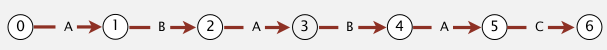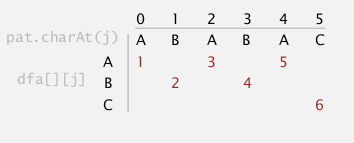• mismatch transition (hard part)

(for j==0, things are simple: `dfa[c]=0` for all `c!=pat`)

• at state `j` (ie. j chars in pattern are matched), and for `c!=pat.charAt(j+1)`
• ⇒ we are in state j: we know the last j chars in input are `pat[0...j-1]`, and followed by char = `c`, so the last j+1 chars of input string is: `pat[0...j-1]+c`
• ⇒ to compute dfa[c][j]: we can simulate as if we backup, ie. `i=i-j+1, j=0`.
• if we go back to set j=0, and set i = i-j+1, then i is pointing at `pat`, the text become `pat[1...j-1]+c`. We then let this string go through our dfa, the state that it achieves is the value of `dfa[c][j]`.

here is a concrete example:
pattern = `"ABABAC"`, state `j=5`, char `c='B`'

• we know the last 6 chars of the input = `pat[0...j-1]+c="ABABA"+"B"="ABABAB"`
• if we backup, i will point to pat, the string is just `pat[1...j-1]+c="BABAB"`
• we use the string "BABAB" as input and go through the partially constructed dfa, and see that we will reach state 4
• so we know `dfa['B']=4`

similarly we can get `dfa['A']=1`, as indicated below:
(pat="ABABAC")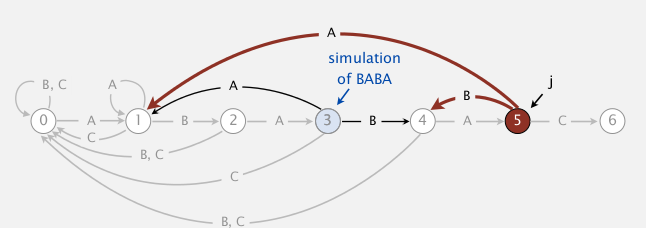one concern: seems this simulation needs `j` steps ?
⇒ can be changed to be constant time if we maintain a state X := the state of simulating of input=pat[1...j-1]
we maintain this state `X`, then for each mismatched char c, we just need to look at `dfa[c][X]`.
(pat="ABABAC")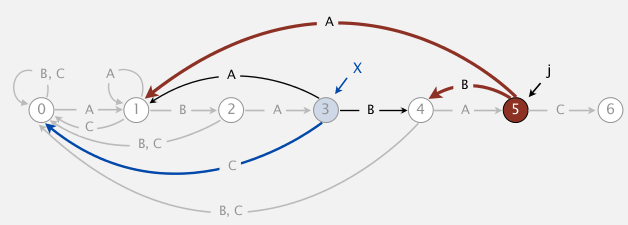[Algo]

• set all matched transitions `dfa[c0][j] = j+1` for all `c0==pat[j]`
• fill first column (j==0): `dfa[c]=0` for all `c!=pat`
• initialize `X=0` (state for empty input string)
• for j=1 to M:
• for all `c!=pat`: set `dfa[c][j] = dfa[c][X]` (DP here...)
• update `X=dfa[c0][X]` ⇒ 注意, 此时X并不等于X+1(最开始`dfa[c0][j]=j+1`不适用于此), 为什么? 因为`c0==pat[j]` 而不`是pat[X]`!! 比如说最开始, j=1的时候X是等于0的!!! (这个弯我饶了好几分钟...)

java code (can be written to be more compate):

```public int[][] constructDFA(String pat){
int R=256;//ASCII code
int M=pat.length();
int[][] dfa = new int[R][M];
// 1. fill matched transitions: dfa[pat.charAt(j)][j] = j+1
for(int j=0;j<M;j++)
dfa[pat.charAt(j)][j] = j+1;
// 2. fill 1st column --> can be ignored as java int default val=0
// 3. fill mismatched transitions
int X = 0;
for(int j=1;j<M;j++){
for(int c=0;c<R;c++)
if(c!=pat.charAt(j))
dfa[c][j] = dfa[c][X];
X = dfa[pat.charAt(j)][X];
}
return dfa;
}
```

running time and space: O(M*R).

prop.
KMP algorithm runs in O(M+N) time, and constructs the dfa in O(M*R) time/space.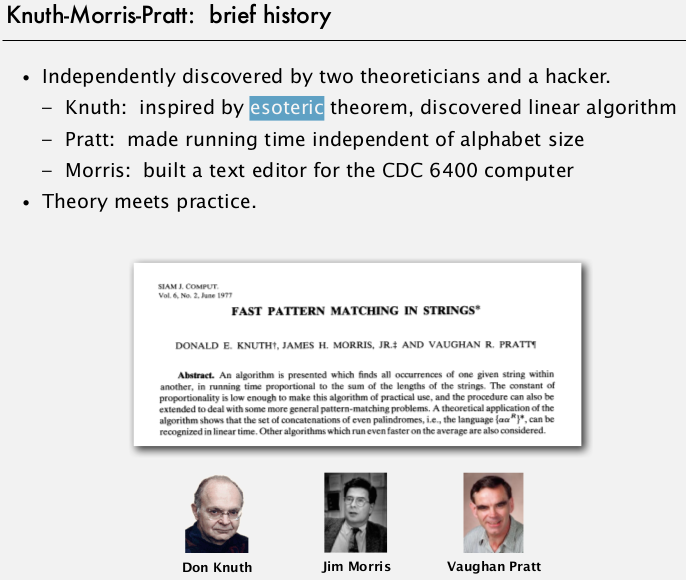# 4. Boyer-Moore

Heuristic in practice.
i does not necessarily go through all txt chars ⇒ i may skip some chars.

### intuition

• for matching: scan chars from right to left (j will decrease when checking)
• when encoutered a mismatch: we can skip <= M chars (if the char is not in pattern)

ex. (pat="NEEDLE")→ pb: how to skip?

### mismatch character heuristic

note: the `i` always points to the beginning of the substring (`txt[i,...,i+M-1]`) to be checked for match.

case 1. mismatched char not in pattern
easy case → just move i to the right of this char.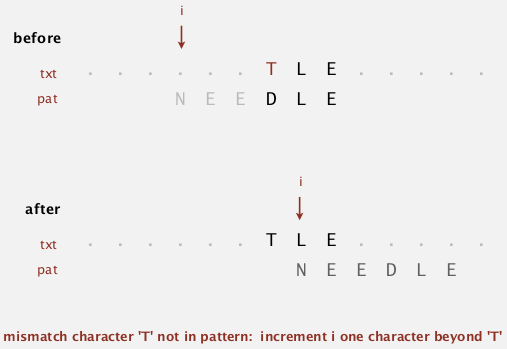case 2. mismatched char in pattern

heuristic: line up i with the rightmost char in pattern.
`i += skip`
where skip length = j - index of rightmost char in pattern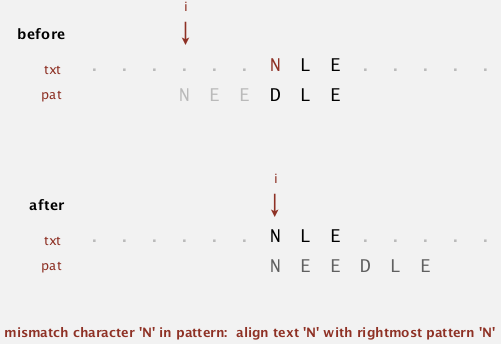note: this does not always help, in the example below, i even backups: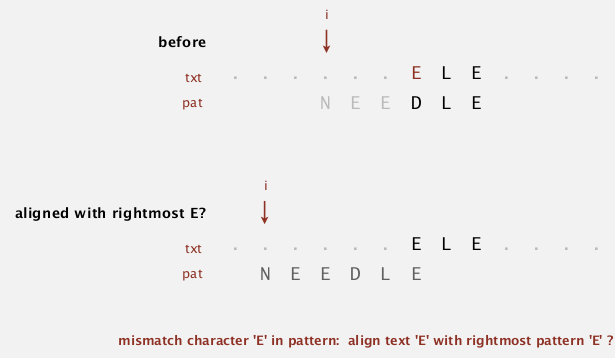to avoid backup, in this case we just increment i by 1 (heuristic doesn't help in this case).

### implementation

use an array `right[]` as skip table, `right[c]` is the index of rightmost occurrence of char c (-1 if c not in pat).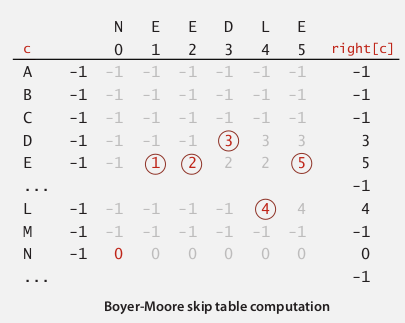```int[] right = new int[M];
for(int i=0;i<R;i++) right[i] = -1;//value for chars not in pattern
for(int j=0;j<M;j++){
right[pat.charAt(j)]=j;
}
```

using this table we can implemente the heuristic algorithm:

```public static int search(String pat, String txt, int[] right){
int N=txt.length(), M=pat.length();
int skip;
for(int i=0;i<N-M;i+=skip){
skip = 0;
for(int j=M-1;j>=0;j++)
if(pat.charAt(j)!=txt.charAt(i+j)){// when mismatch happens
skip = Math.max(1,j - right[txt.charAt(i+j)]);// skip if we can, else just increment i by 1
break;
}
if(skip==0)// if the above for loop finishes without changing skip --> we are done.
return i;
}
return N;// pattern not found
}
```

### analysis

property. the Boyer-Moore heuristic (in practice) takes about N/M (sublinear!) compares to search.

worst-case performance: N*M... 这一点不如KMP.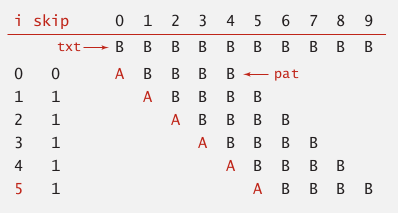→ can be improved...

# 5. Rabin-Karp

### intuition

basic idea: modular hashing
ex. for strings of numbers

• compute hash fcn (for number strings is easy: take the string and treat it as a number, then %Q where Q is a big prime number).
• for a pointer i →corresponds to the substring `txt[i, ..., i+M-1]` → check hash for match

(below: text=3141592653589793, pattern=26535)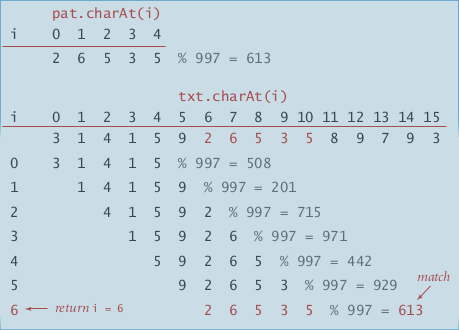### computing the hash function efficiently

let ti be the ith char in txt, the hashcode for substring `txt[i,...,i+M-1]` is:⇒ just an M-digit base-R integer modulo Q ! `poly(M, R) % Q`*. *

• Honor's method

linear time algorithm for evaluating polynomial.
recursive equation: `poly(i, R) = poly(i-1, R)*R+ti`

ex. (R=10, M=5)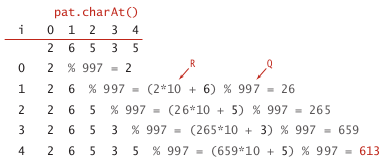```private long hash(String key, int M){
long h=0;
for(int i=0;i<M;i++)
h = ( h*R + key.charAt(i) ) % Q
return h;
}
```
• if we know x_i, the x_i+1 can be infered: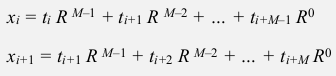⇒ x_i+1 can be computed in constant time: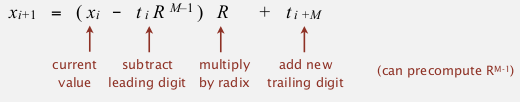⇒ we precompute R^(M-1) and maintain the hash number, and check for match !

```public static int search(String txt, String pat){
int N=txt.length(), M=pat.length();
long pathash = hash(pat, M);
int RM = R^(M-1);// <-- pseudo code, store value of R^(M-1)
long txthash = hash(txt, M);// txthash will be maintained
for(int i=0;i<N-M;i++){
if(txthash==pathash && checkMatch(i,txt,pat))
return i;
txthash = ( (txthash - txt.charAt(i)*RM)*R + txt.charAt(i+M) ) % Q;
}
}
```

for collisions: Monte Carlo vs. Las Vegas

### analysis

Theory: if Q is sufficiently large (~M*N^2), the probability of collision is ~1/N.
Practice: choose Q to be sufficiently large, and collision probability is ~1/Q.# Summery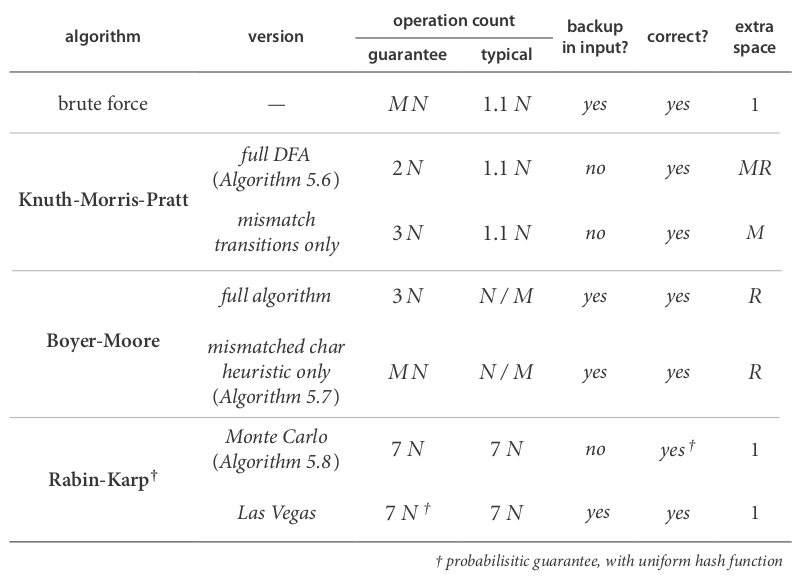#### Part 8 of series «Algorithms Princeton MOOC II»：

comments powered by Disqus